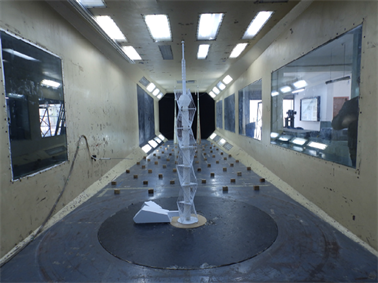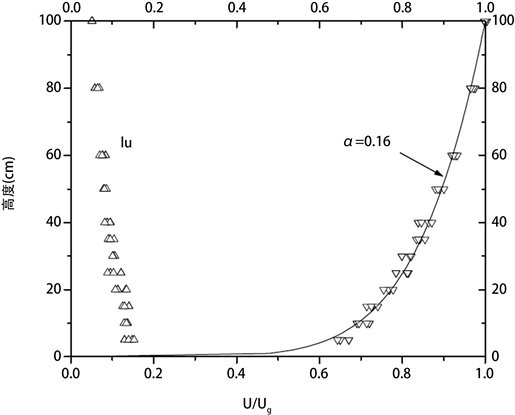#### 期刊菜单

Study on Wind-Induced Effect of Wind Sensitive High-Rise Steel Structure TV Tower
DOI: 10.12677/HJCE.2022.114062, PDF , HTML, XML, 下载: 150  浏览: 203

Abstract: The high-rise steel structure TV tower has the characteristics of high structural height and relatively small structural stiffness and is highly sensitive to wind load. In this paper, the steel structure TV tower adopts the rigid model with reduced scale, carries out the pressure measurement wind tunnel test of rigid model group buildings, and measures the wind pressure value on the surface of the TV tower Model under 360˚ wind angle, so that the average wind pressure value, fluctuating wind pressure value and wind load shape coefficient on the physical surface of the TV tower can be calculated correctly to determine the most unfavorable wind direction angle and the wind vibration coefficient varying along with the height of the TV tower. The wind-induced vibration response of the structure is analyzed according to the test. The fluctuating pressure-time history of each measuring point obtained from the wind tunnel test of the rigid body model is applied to the finite element model of structural dynamic analysis. The frequency-domain analysis of the structure is carried out to determine the wind-induced vibration displacement and acceleration response of its important parts and the equivalent wind load of each floor, so as to provide a necessary and reliable basis for the design.

1. 引言

2. 工程概况

3. 验概况

3.1. 试验风洞

3.2. 试验模型Figure 1. Wind tunnel pressure measurement model and turntableFigure 2. Average wind speed and turbulence profile of wind field simulating class B atmospheric boundary layer

4. 结构设计的风洞试验结果分析

4.1. 不同风向角下各测点的平均风压系数

${C}_{Pi,r}=\frac{{P}_{i}-{P}_{rs}}{{P}_{r0}-{P}_{rs}}=\frac{{P}_{i}-{P}_{rs}}{\frac{1}{2}\rho {U}_{r}^{2}}$ (1)

${C}_{Pi,r}=\frac{{P}_{iu}-{P}_{id}}{\frac{1}{2}\rho {U}_{r}^{2}}$ (2)

${C}_{P}={\left({Z}_{r}/{H}_{G}\right)}^{2\alpha }{C}_{P,r}$ (3)

${C}_{P}={\left(50/350\right)}^{0.15}{C}_{P,r}=0.7468{C}_{P,r}$ (4)

4.2. 不同风向角下各测点的局部体型系数和层体型系数

《建筑结构荷载规范》(GB 50009-2012)中规定的作用在建筑物表面上高度Z处的风荷载标准值的计算公式为：

$\omega ={\beta }_{z}{\mu }_{s}{\mu }_{z}{\omega }_{0,R}$ (5)

${\mu }_{si}={C}_{Pmean,i}×{\left(\frac{350}{z}\right)}^{0.30}$ (6)

${\mu }_{s,bX}=\frac{{\sum }_{i=1}^{n}{\mu }_{si}{L}_{i}{n}_{X}}{{D}_{X}}$ (7a)

${\mu }_{s,bY}=\frac{{\sum }_{i=1}^{n}{\mu }_{si}{L}_{i}{n}_{Y}}{{D}_{Y}}$ (7b)

5. 围护结构设计的风压试验结果分析

5.1. 围护结构设计风压计算方法

5.2. 风压的极值统计确定方法

${C}_{\text{Pmax}}={C}_{\text{Pmean}}+k{C}_{\text{Prms}}$ (8a)

${C}_{\text{Pmin}}={C}_{\text{Pmean}}-k{C}_{\text{Prms}}$ (8b)

6. 电视塔结构风振响应分析

6.1. 结构风致响应分析方法Table 1. Structural wind-induced response analysis parameters

$\left\{{t}_{k}\right\}=\left\{{t}_{k,m}\right\}/{\lambda }_{t}$ (9)

6.2. 10年重现期风振分析结果

6.3. 50年和100年重现期风振分析结果Table 2. Total internal force of overall section at the bottom of TV tower at main wind direction angleTable 3. Shape coefficient of main structure of TV tower at main wind direction angle

6.4. 风洞实验结果与规范对比分析Table 4. Comparison of internal force between wind tunnel test and code

7. 结论

1、按照现行的建筑结构荷载规范及国内外研究进展，本文提供了两种方法计算的围护结构设计用风荷载，即规范提供的阵风风荷载和极值风荷载。在实际应用时，建议偏保守地取极值风荷载作为用于围护结构设计用风荷载。

2、基于试验得到的体形系数，计算的10分钟平均风荷载(50年或100年重现期)作为结构设计的基本参数。在此基础上，通过结构风振分析给出了高耸结构各层的平均风荷载和抖振等效风荷载，可供结构整体设计使用，其中结构风振响应分析中已考虑顺风向和横风向风致振动效应。

3、电视塔139.4米以下主体结构部分的等效体型系数(参考宽度为19米)在来流风向角为180度时取得最大值0.601。139.4米以上天线部分的体型系数(以天线在体轴方向上的投影宽度为参考宽度)为0.60。

4、根据风洞实验与按规范计算所得风荷载作用下结构基底剪力及基底倾覆弯矩，规范计算结果均小于风洞实验结果，因此结构设计时采用风洞实验报告提供的风荷载参数可作为风荷载输入的依据。

  中华人民共和国住房和城乡建设部. 钢结构设计标准: GB 50017-2017 [S]. 北京: 中国建筑工业出版社, 2017.  中华人民共和国住房和城乡建设部. 建筑抗震设计规范: GB 50011-2010 [S]. 北京: 中国建筑工业出版社, 2016.  中华人民共和国住房和城乡建设部. 高耸结构设计标准: GB 50135-2019 [S]. 北京: 中国建筑工业出版社, 2019.  马人乐. 钢塔的非线性动力分析[J]. 建筑结构学报, 1986(3): 45-56.  李君, 张耀春. 高层钢结构的非线性动力全过程分析方法[J]. 哈尔滨建筑大学学报, 2000, 33(1): 16-19.  任志刚, 李培鹏, 王乾坤. 复杂高耸钢结构电视塔风振响应分析[J]. 工业建筑, 2016, 46(6): 137-143.  禹慧, 马人乐, 何敏娟. 复杂高耸结构三维风振响应时程分析[J]. 特种结构, 2007, 24(4): 45-49.  郅伦海, 毛硕. 高耸结构风荷载特性的风洞试验研究[J]. 武汉理工大学学报, 2018, 40(1): 35-42.  李秋胜, 李慧真, 李毅. 椭圆形高耸结构风荷载特性试验研究[J]. 湖南大学学报(自然科学版), 2015, 42(1): 1-8.  华坤, 彭益成, 陈轩, 等. 独立式避雷针风振响应分析及结构优化[J]. 建筑结构, 2021, 51(S1): 1789-1793.  王世村, 孙炳楠, 楼文娟. 单杆输电塔气弹模型风洞试验研究和理论分析[J]. 浙江大学学报(工学版), 2005, 39(1): 87-91.